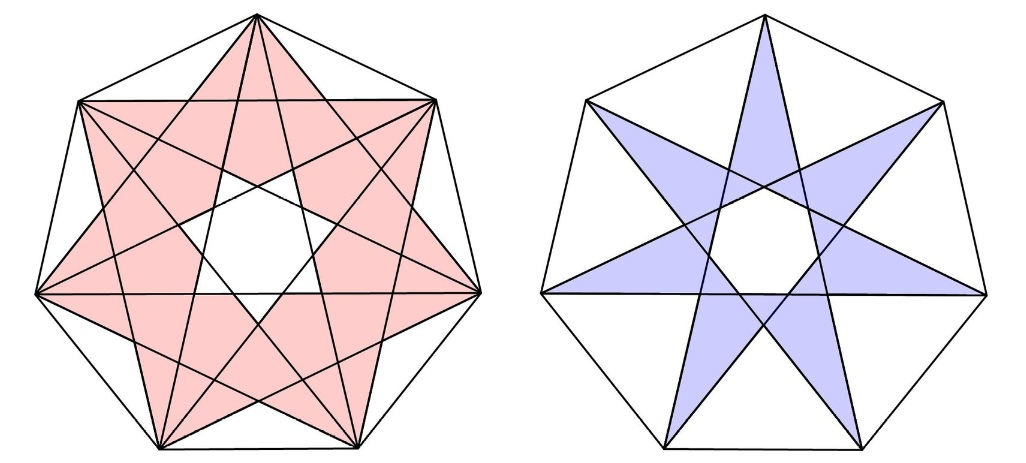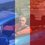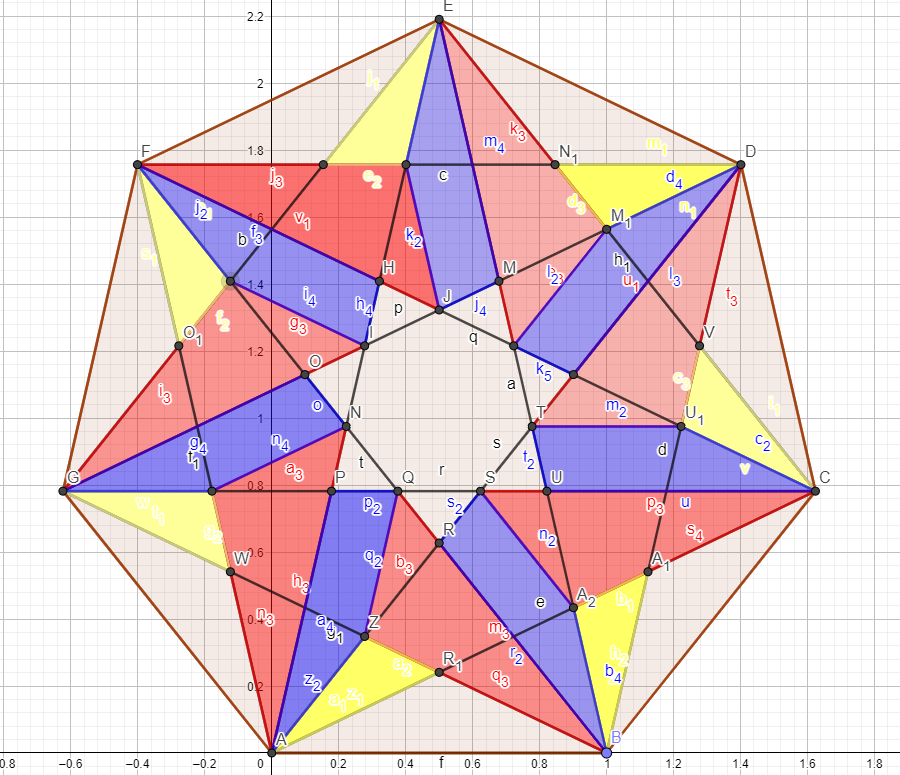# Analytic solution for areas ratioFind the ratio of the red area to the blue area. Regular heptagons are equal.

Inspiration1

Inspiration2 -Septic Triangle

Phyton give 2.

  1 2 3 4 5 6 7 8 9 10 11 12 13 14 15 16 17 18 19 20 21 22 23 24 25 26 27 28 29 30 31 32 33 34 35 36 37 38 39 40 41 42 43 44 from math import isclose from sympy import * radius = csc(pi / 7) / 2 # arrange for side length = 1 center = Point(0, 0) # centered at Origin p = Polygon(center, radius, n=7) side = trigsimp(p.sides.length) assert (isclose(side, 1, abs_tol=1e-30)) A, B, C, D, E, F, G = p.vertices print(A) print(B) AE = Line(A, E) GC = Line(G, C) BF = Line(B, F) GB = Line(G, B) FA = Line(F, A) P = FA.intersection(GB) H = AE.intersection(GC) J = AE.intersection(BF) I = GC.intersection(BF) area = Triangle(H, I, J).area*7 Big_area = Triangle(Point(0,0),A,B).area*7 Lit_area = Triangle(Point(0,0),J,I).area*7 SideL_area = Triangle(G,A,P).area*7 SideB_area = Triangle(G,A,H).area*7 S= Big_area-SideL_area-Lit_area-area s= Big_area-SideB_area-Lit_area print(S/s) S_area = N(S, 20) s_area =N(s,20) print(S_area) print(s_area) print(S_area/s_area) (-7*(-9 + cos(pi/14)/sin(pi/7) + 4*cos(3*pi/14)/sin(pi/7))/(32*(sin(pi/14) + 1)*sin(pi/14)*sin(pi/7)) - 7*(1 - sin(3*pi/14))*(-cos(3*pi/14) + cos(pi/14))/(4*(-sin(pi/7) + sin(2*pi/7) + cos(pi/14))*sin(pi/7)) - 7*(-cos(3*pi/14) + cos(pi/14))*(-789*cos(2*pi/7)/(8*sin(pi/7)) - 789*sin(3*pi/14)/(8*sin(pi/7)) - 385*sin(pi/14)/(4*sin(pi/7)) - 15*sin(5*pi/14)/(4*sin(pi/7)) - 15*(1 - cos(2*pi/7))**2*cos(2*pi/7)/sin(pi/7) - 23*(1 - cos(2*pi/7))**2*sin(pi/14)/sin(pi/7) - 3*(1 - cos(2*pi/7))**2*sin(3*pi/14)/sin(pi/7) + 9*(1 - cos(pi/7))**2/(2*sin(pi/7)) + 43*cos(3*pi/7)/(8*sin(pi/7)) + 2*(1 - cos(3*pi/7))**2/sin(pi/7) + 35*(1 - cos(2*pi/7))**2/(4*sin(pi/7)) + 10*(1 - cos(2*pi/7))**2/tan(pi/7) + 36/tan(pi/7) + 901/(8*sin(pi/7)))/(32*(sin(2*pi/7) + 2*sin(pi/7))*(-sin(pi/7) + sin(2*pi/7) + cos(pi/14))*(-6*cos(pi/14) + 5*sin(pi/7) + 4*cos(3*pi/14))*sin(pi/14)*sin(pi/7)**2) + 7*sin(2*pi/7)*csc(pi/7)**2/8)/(-7*(-48*sin(pi/7) - 22*cos(5*pi/14) - 7*sin(2*pi/7) + 35*cos(pi/14))/(4*(-60*cos(2*pi/7) - 49*sin(3*pi/14) - 75*sin(pi/14) - 20*sin(2*pi/7)**2 - 6*cos(pi/7) - 8*cos(2*pi/7)*cos(3*pi/7) + 8*cos(3*pi/7) + 38*sin(5*pi/14) + 66)) - 7*(1 - sin(3*pi/14))*(-cos(3*pi/14) + cos(pi/14))/(4*(-sin(pi/7) + sin(2*pi/7) + cos(pi/14))*sin(pi/7)) + 7*sin(2*pi/7)*csc(pi/7)**2/8) 2.4356342034533018176 1.2178171017266509088 2.0000000000000000000Note by Yuriy Kazakov
4 months, 3 weeks ago

This discussion board is a place to discuss our Daily Challenges and the math and science related to those challenges. Explanations are more than just a solution — they should explain the steps and thinking strategies that you used to obtain the solution. Comments should further the discussion of math and science.

When posting on Brilliant:

• Use the emojis to react to an explanation, whether you're congratulating a job well done , or just really confused .
• Ask specific questions about the challenge or the steps in somebody's explanation. Well-posed questions can add a lot to the discussion, but posting "I don't understand!" doesn't help anyone.
• Try to contribute something new to the discussion, whether it is an extension, generalization or other idea related to the challenge.

MarkdownAppears as
*italics* or _italics_ italics
**bold** or __bold__ bold
- bulleted- list
• bulleted
• list
1. numbered2. list
1. numbered
2. list
Note: you must add a full line of space before and after lists for them to show up correctly
paragraph 1paragraph 2

paragraph 1

paragraph 2

[example link](https://brilliant.org)example link
> This is a quote
This is a quote
    # I indented these lines
# 4 spaces, and now they show
# up as a code block.

print "hello world"
# I indented these lines
# 4 spaces, and now they show
# up as a code block.

print "hello world"
MathAppears as
Remember to wrap math in $$ ... $$ or $ ... $ to ensure proper formatting.
2 \times 3 $2 \times 3$
2^{34} $2^{34}$
a_{i-1} $a_{i-1}$
\frac{2}{3} $\frac{2}{3}$
\sqrt{2} $\sqrt{2}$
\sum_{i=1}^3 $\sum_{i=1}^3$
\sin \theta $\sin \theta$
\boxed{123} $\boxed{123}$

Sort by:

As simple as that - we cut the big star into 21 pieces and make up two identical stars - one of the red and the second of the yellow and blue pieces.- 4 months, 2 weeks ago

That's...incredible. Simply amazing Yuriy!

- 4 months, 2 weeks ago

Sympy! Cool!

- 4 months, 2 weeks ago

Yeah, sympy is fun to play with, isn't it? I wish it were a little better at simplifying trig expressions, though. That one is frightening me. :)

- 4 months, 2 weeks ago

Problem - find solution without Sympy. Only paper and pencil.

- 4 months, 2 weeks ago

You should tweet this to Cshearer41 on Twitter with a caption of

"Prove that the area of the pink region is twice the area of the blue region"

- 4 months, 2 weeks ago